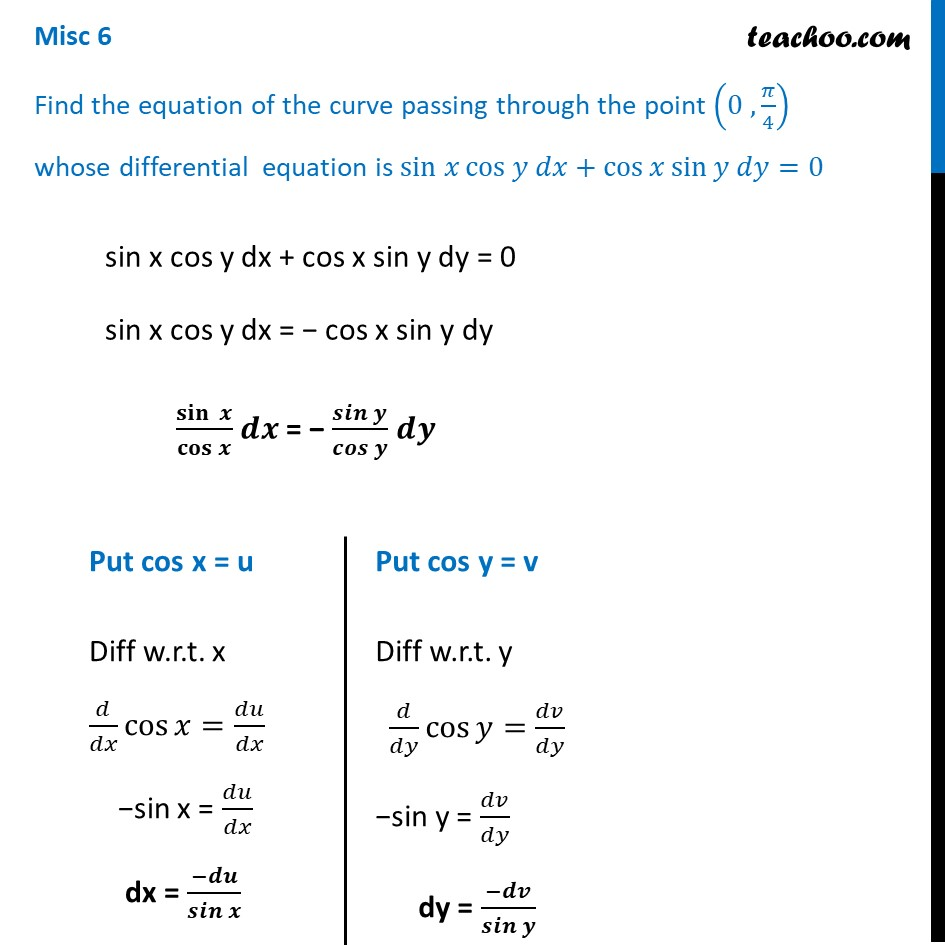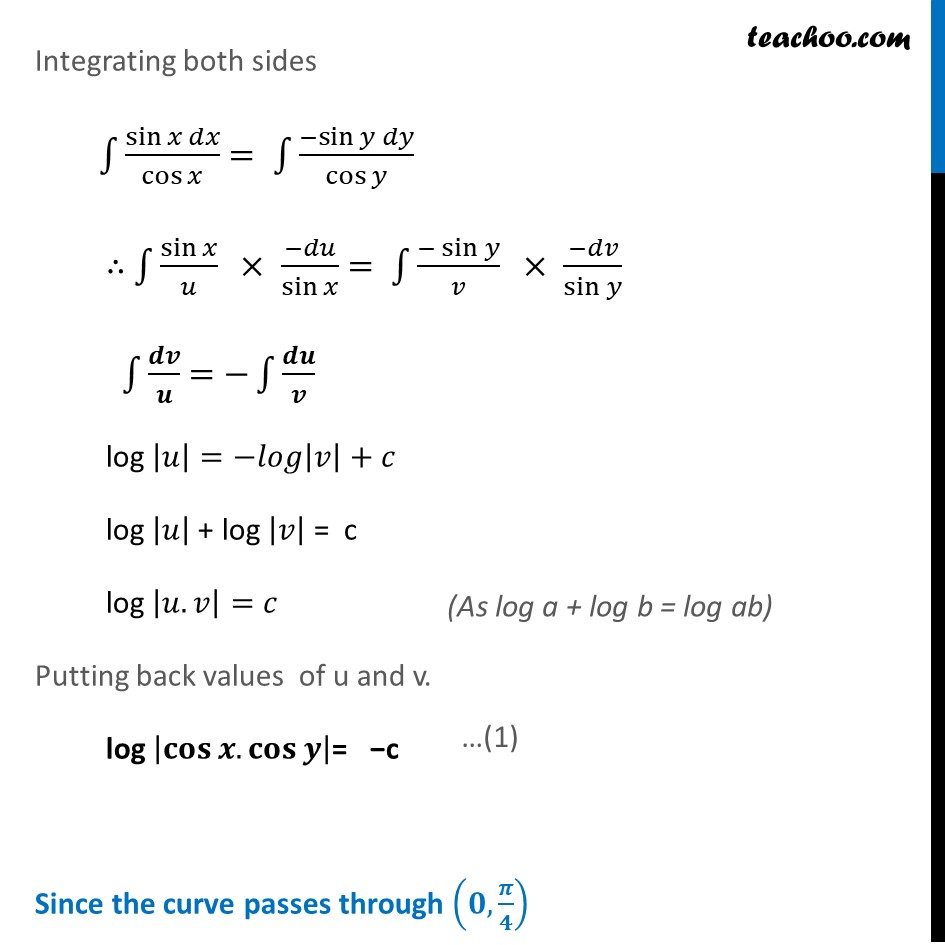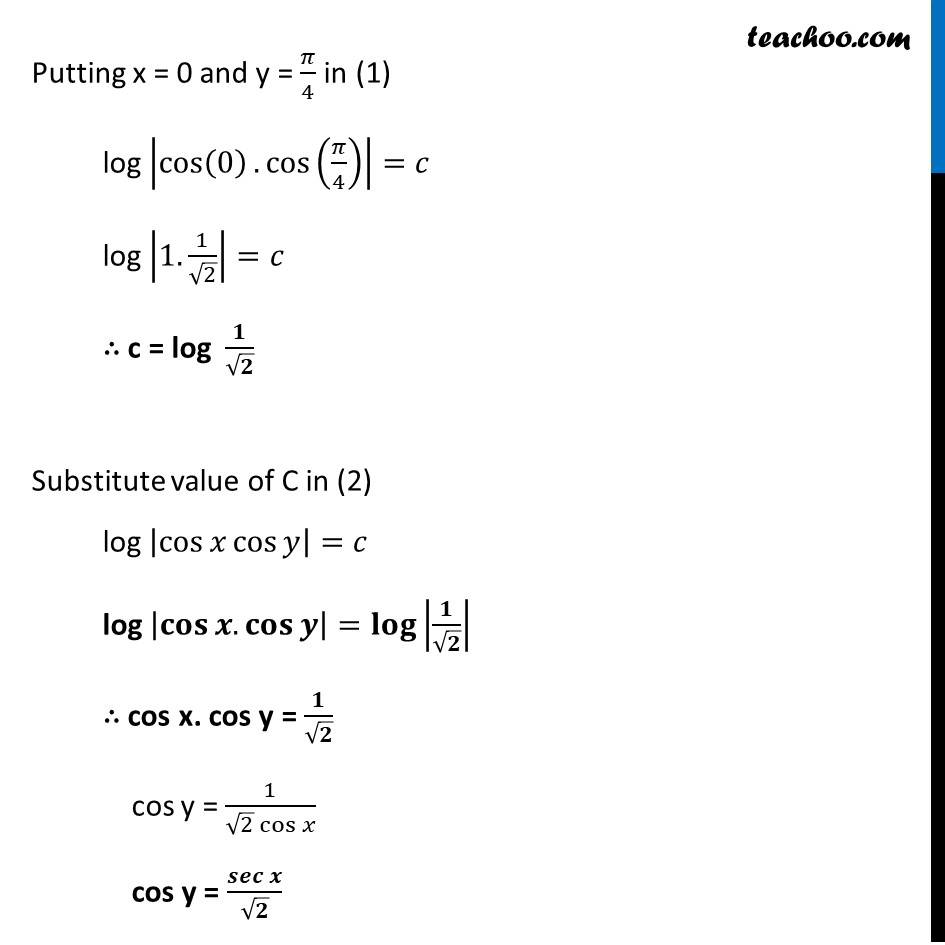Miscellaneous

Chapter 9 Class 12 Differential Equations
Serial order wiseLearn in your speed, with individual attention - Teachoo Maths 1-on-1 Class

### Transcript

Misc 6 Find the equation of the curve passing through the point (0 , 𝜋/4) whose differential equation is sin 𝑥 cos⁡〖𝑦 𝑑𝑥+cos⁡〖𝑥 sin⁡〖𝑦 𝑑𝑦=0〗 〗 〗 sin x cos y dx + cos x sin y dy = 0 sin x cos y dx = − cos x sin y dy 〖𝐬𝐢𝐧 〗⁡𝒙/𝐜𝐨𝐬⁡𝒙 𝒅𝒙 = − 𝒔𝒊𝒏⁡𝒚/𝒄𝒐𝒔⁡𝒚 𝒅𝒚 Put cos x = u Diff w.r.t. x 𝑑/𝑑𝑥 cos⁡〖𝑥=𝑑𝑢/𝑑𝑥 〗 −sin x = 𝑑𝑢/𝑑𝑥 dx = (−𝒅𝒖)/𝒔𝒊𝒏⁡𝒙 Put cos y = v Diff w.r.t. y 𝑑/𝑑𝑦 cos⁡〖𝑦=𝑑𝑣/𝑑𝑦 〗 −sin y = 𝑑𝑣/𝑑𝑦 dy = (−𝒅𝒗)/𝒔𝒊𝒏⁡𝒚 Integrating both sides ∫1▒sin⁡〖𝑥 𝑑𝑥〗/cos⁡𝑥 = ∫1▒〖−sin〗⁡〖𝑦 𝑑𝑦〗/cos⁡𝑦 ∴ ∫1▒〖sin⁡𝑥/𝑢 × (−𝑑𝑢)/sin⁡𝑥 〗= ∫1▒〖(−sin⁡𝑦)/𝑣 × (−𝑑𝑣)/sin⁡𝑦 〗 ∫1▒𝒅𝒗/𝒖=−∫1▒𝒅𝒖/𝒗 log |𝑢|=−𝑙𝑜𝑔|𝑣|+𝑐 log |𝑢| + log |𝑣| = c log |𝑢.𝑣|=𝑐 Putting back values of u and v. log |𝐜𝐨𝐬⁡〖𝒙.𝐜𝐨𝐬⁡𝒚 〗 |= −c Since the curve passes through (𝟎, 𝝅/𝟒) Putting x = 0 and y = 𝜋/4 in (1) log |cos⁡(0).cos⁡(𝜋/4)|=𝑐 log |1. 1/√2|=𝑐 ∴ c = log 𝟏/√𝟐 Substitute value of C in (2) log |cos⁡〖𝑥 cos⁡𝑦 〗 |=𝑐 log |𝐜𝐨𝐬⁡〖𝒙.𝐜𝐨𝐬⁡𝒚 〗 |=𝐥𝐨𝐠⁡|𝟏/√𝟐| ∴ cos x. cos y = 𝟏/√𝟐 cos y = 1/(√2 cos⁡𝑥 ) cos y = (𝒔𝒆𝒄 𝒙)/√𝟐